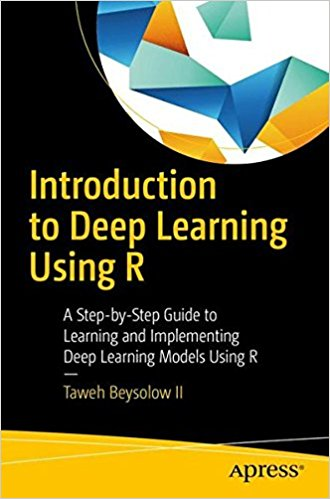# Introduction to Deep Learning Using R – pdf – 电子书免费下载Understand deep learning, the nuances of its different models, and where these models can be applied.

The abundance of data and demand for superior products/services have driven the development of advanced computer science techniques, among them image and speech recognition. Introduction to Deep Learning Using Rprovides a theoretical and practical understanding of the models that perform these tasks by building upon the fundamentals of data science through machine learning and deep learning. This step-by-step guide will help you understand the disciplines so that you can apply the methodology in a variety of contexts. All examples are taught in the R statistical language, allowing students and professionals to implement these techniques using open source tools.

What You’ll Learn

• Understand the intuition and mathematics that power deep learning models
• Utilize various algorithms using the R programming language and its packages
• Use best practices for experimental design and variable selection
• Practice the methodology to approach and effectively solve problems as a data scientist
• Evaluate the effectiveness of algorithmic solutions and enhance their predictive power
Who This Book Is For

Students, researchers, and data scientists who are familiar with programming using R. This book also is also of use for those who wish to learn how to appropriately deploy these algorithms in applications where they would be most useful.

ISBN:1484227336

## 锦瑟无端五十弦，一弦一柱思华年

### 觉得文章有用就打赏一下文章作者

#### 支付宝扫一扫打赏#### 微信扫一扫打赏